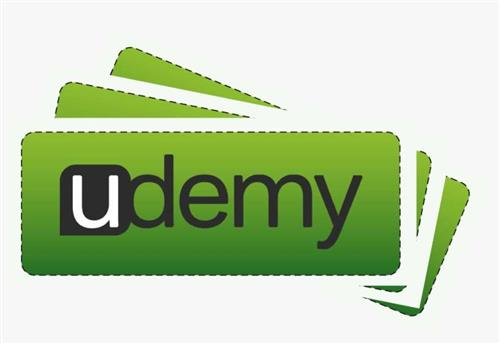# Udemy - Recursion, Backtracking and Dynamic Programming in Python110

#### TOP

##### Alpha and Omega
Member
AccessMP4 | Video: h264, 1280x720 | Audio: AAC, 44.1 KHz, 2 Ch
Genre: eLearning | Language: English + srt | Duration: 91 lectures (9h 9m) | Size: 1.33 GB
Learn Recursion, Backtracking, Divide and Conquer Methods and Dynamic programming via Examples and Problems in Python

What you'll learn:
Understanding recursion
Understand backtracking
Understand dynamic programming
Understand divide and conquer methods
Implement 15+ algorithmic problems from scratch
Improve your problem solving skills and become a stronger developer
Requirements
Basic Python
Description
This course is about the fundamental concepts of algorithmic problems focusing on recursion, backtracking, dynamic programming and divide and conquer approaches. As far as I am concerned, these techniques are very important nowadays, algorithms can be used (and have several applications) in several fields from software engineering to investment banking or R&D.
Section 1 - RECURSION
what are recursion and recursive methods
stack memory and heap memory overview
what is stack overflow?
Fibonacci numbers
factorial function
tower of Hanoi problem
Section 2 - SEARCH ALGORITHMS
linear search approach
binary search algorithm
Section 3 - SELECTION ALGORITHMS
what are selection algorithms?
how to find the k-th order statistics in O(N) linear running time?
quickselect algorithm
median of medians algorithm
the secretary problem
Section 4 - BACKTRACKING
what is backtracking?
n-queens problem
Hamiltonian cycle problem
coloring problem
knight's tour problem
maze problem
Section 5 - DYNAMIC PROGRAMMING
what is dynamic programming?
knapsack problem
rod cutting problem
subset sum problem
Section 6 - OPTIMAL PACKING
what is optimal packing?
bin packing problem
Section 7 - DIVIDE AND CONQUER APPROACHES
what is the divide and conquer approach?
dynamic programming and divide and conquer method
how to achieve sorting in O(NlogN) with merge sort?
the closest pair of points problem
In each section we will talk about the theoretical background for all of these algorithms then we are going to implement these problems together from scratch in Python.
Thanks for joining the course, let's get started!
Who this course is for
This course is meant for newbies who are not familiar with algorithmic problems in the main or students looking for some refresher
Anyone preparing for programming interviews or interested in improving their problem solving skills
Homepage
Code:

A 0

Member
Thank you!

K 0

#### kolizaer

##### Transcendent
MemberMP4 | Video: h264, 1280x720 | Audio: AAC, 44.1 KHz, 2 Ch
Genre: eLearning | Language: English + srt | Duration: 91 lectures (9h 9m) | Size: 1.33 GB
Learn Recursion, Backtracking, Divide and Conquer Methods and Dynamic programming via Examples and Problems in Python

What you'll learn:
Understanding recursion
Understand backtracking
Understand dynamic programming
Understand divide and conquer methods
Implement 15+ algorithmic problems from scratch
Improve your problem solving skills and become a stronger developer
Requirements
Basic Python
Description
This course is about the fundamental concepts of algorithmic problems focusing on recursion, backtracking, dynamic programming and divide and conquer approaches. As far as I am concerned, these techniques are very important nowadays, algorithms can be used (and have several applications) in several fields from software engineering to investment banking or R&D.
Section 1 - RECURSION
what are recursion and recursive methods
stack memory and heap memory overview
what is stack overflow?
Fibonacci numbers
factorial function
tower of Hanoi problem
Section 2 - SEARCH ALGORITHMS
linear search approach
binary search algorithm
Section 3 - SELECTION ALGORITHMS
what are selection algorithms?
how to find the k-th order statistics in O(N) linear running time?
quickselect algorithm
median of medians algorithm
the secretary problem
Section 4 - BACKTRACKING
what is backtracking?
n-queens problem
Hamiltonian cycle problem
coloring problem
knight's tour problem
maze problem
Section 5 - DYNAMIC PROGRAMMING
what is dynamic programming?
knapsack problem
rod cutting problem
subset sum problem
Section 6 - OPTIMAL PACKING
what is optimal packing?
bin packing problem
Section 7 - DIVIDE AND CONQUER APPROACHES
what is the divide and conquer approach?
dynamic programming and divide and conquer method
how to achieve sorting in O(NlogN) with merge sort?
the closest pair of points problem
In each section we will talk about the theoretical background for all of these algorithms then we are going to implement these problems together from scratch in Python.
Thanks for joining the course, let's get started!
Who this course is for
This course is meant for newbies who are not familiar with algorithmic problems in the main or students looking for some refresher
Anyone preparing for programming interviews or interested in improving their problem solving skills
Homepage
Code:

* Hidden text: cannot be quoted. *

thx for sharing thisReplies
1
Views
23
KatzSec DevOps
KReplies
1
Views
32
KatzSec DevOps
KReplies
1
Views
32
KatzSec DevOps
KReplies
0
Views
74
TOPReplies
0
Views
56
TOP